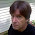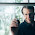## Thursday, October 21, 2010

### Electromagnetism, photons, and symmetry

After this rather abstract enumeration, it is time to take a closer look at a particular example. The simplest sector embedding a local symmetry in the standard model is electromagnetism. Classically, electromagnetism describes electric and magnetic fields, and thus also light, X-rays, and every other form of electromagnetic waves. As their names already hints, electric and magnetic fields are so-called fields. Fields in physics are something which associate with each point in space and with each instance in time a quantity. In case of electromagnetism this is a quantity describing the electric and magnetic properties at this point. Each of these two properties turn out to have a strength and a direction. Thus the electric and magnetic fields associate with each point in space and time an electric and a magnetic magnitude and a direction. For a magnetic field this is well known from daily experience. Go around with a compass. As you move, the magnetic needle will arrange itself in response to the geomagnetic field. Thus, this demonstrates that there is a direction involved with magnetism. That there is also a strength involved you can see when moving two magnets closer and closer together. How much they pull at each other depends on where they are relative to each other. Thus there is also a magnitude associated with each point. The same actually applies to electric fields, but this is not as directly testable with common elements. Ok, so it is now clear that electric and magnetic fields have a direction and a magnitude. Thus, at each point in space and time six numbers are needed to describe them: two magnitudes and two angles each to determine a direction.

When in the 19th century people tried to understand how electromagnetism works they also figured this out. However, they made also another intriguing discovery. When writing down the laws which govern electromagnetism, it turns out that electric and magnetic fields are intimately linked, and that they are just two sides of the same coin. That is the reason to call it electromagnetism. In the early 20th century it then became clear that both phenomena can be associated with a single particle, the photon. But then it was found that to characterize a photon only two numbers at each point in space and time are necessary. This implies that between the six numbers characterizing electric and magnetic fields relations exist. These are known as Maxwell equations in classical physics, or as quantum Maxwell dynamics in the quantum theory. If you would add, e. g., electrons to this theory, you would end up with quantum electro dynamics - QED.

So, this appeared as a big step forward in describing numerically electromagnetism. However, when looking deeper into the mathematical concepts, it turned out to be technically rather complicated to describe all electric and magnetic phenomena with just these two properties of the photon. It was then that people noticed that including a certain redundancy things became much simpler. An ideal solution was found to describe electromagnetism with four numbers at each space-time point, instead of two. These can then not be independent, of course. And it is here where the symmetry comes into play: It is a symmetry concept which connects these numbers.

First, here is a simple example of how it works. Take someone walking only along the circumference of a circle. Then you can either describe her position by the height and width from the center of the circle. Or you can use the angle around the circle's circumference. Both is equally valid. Hence, the two numbers of the first choice are uniquely connected to the second choice: Changing the angle will change both height and width simultaneously! And because this connection comes from the fact that the circle is rotationally symmetric, it is this symmetry. And the symmetry of a circle is called U(1). Now, the relation between the four convenient numbers and the two important ones is quite in analogy to this case, and is therefore also a U(1) symmetry. That is how the symmetry becomes associated with electromagnetism. This tells us that if we change the four numbers by, so to say, moving them around on the circle, we do not change the two numbers describing the photon (or the six describing the electric and magnetic field). Only when we move away from the circumference, the two (and six) numbers change. In this way the symmetry is only helping us in a mathematical description, but is not influencing what we can measure. It is therefore also called a gauge symmetry. It is actually a local gauge symmetry, because these are fields, and we can do this at every point.

1.You don't explicitly state what the fourth number is. I'm guessing it's the radius?

2.In a certain sense, yes. However, the analogy with a circle is just an analogy, and the four numbers in truth correspond to a more complicated mathematical object, a so-called four-vector, which changes very specifically under such a symmetry transformation.

3.I have discussed this a bit more in the latest post.

4.Well, thank you very much, Alex. I love this blog of yours and what you are doing. keep up the good work. :-)

5.Thank you - and sorry for having not written something in a while. It has been quite busy. I hope that it finally gets better.

6.This post helped me alot. Thanks for sharing with us!
Find more about Electromagnetic Field Theory